## Solution to 1986 Problem 1

 (A) The acceleration of the rock is always equal to the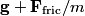$\mathbf{g} + \mathbf{F}_{\text{fric}}/m$ where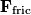$\mathbf{F}_{\text{fric}}$ is the friction force.(B) As stated above, the acceleration of the rock is always equal to the$\mathbf{g} + \mathbf{F}_{\text{fric}}/m$ where$\mathbf{F}_{\text{fric}}$ is the friction force. When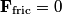$\mathbf{F}_{\text{fric}} = 0$, then the acceleration of the rock is just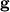$\mathbf{g}$. Therefore, (B) is the correct answer.(C)$\mathbf{F}_{\text{fric}}$ is in the same direction as$\mathbf{g}$ on the way up, and in the opposite direction on the way down.(D) Part of the rock's initial kinetic energy is dissipated as heat during its flight, therefore, the kinetic energy of the rock upon return to its starting point must be less than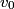$v_0$. (E) By conservation of energy, the rock can never have a kinetic energy greater than its initial kinetic at any point during its flight.